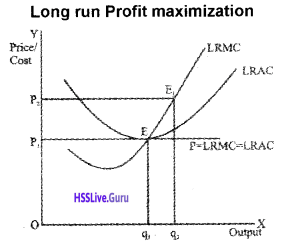# Plus Two Economics Chapter Wise Questions and Answers Chapter 4 The Theory of The Firm Under Perfect Competition

Students can Download Chapter 4 The Theory of The Firm Under Perfect Competition Questions and Answers, Plus Two Economics Chapter Wise Questions and Answers helps you to revise the complete Kerala State Syllabus and score more marks in your examinations.

## Kerala Plus Two Economics Chapter Wise Questions and Answers Chapter 4 The Theory of The Firm Under Perfect Competition

### Plus Two Economics The Theory of The Firm Under Perfect Competition One Mark Questions and Answers

Question 1.
TR curve under perfect competition passes through the origin. Do you agree?
Yes, I agree.

Question 2.
Pick the odd one out.

1. Homogeneous products, free entry, and exit, price maker.
2. Homogeneous product, freedom of entry and exit, large number of buyers and sellers, product differentiation.
3. MC = MR, MR = AFC, MC cuts MR from below, P = AC.

1. Price maker. Others are features of perfect competition.
2. Product differentiation. Others are features of perfect competition.
3. MR = AFC. Others are conditions for firm’s equilibrium under perfect competition.

Question 3.
Pick out the odd one and justify it.
Free entry, profit maximization, perfect knowledge, price discrimination.
Price discrimination. Others are features of perfect competition.

Question 4.
Which of the following represents normal profit?
(a) MR = MC
(b) AR = AC
(c) TR > TC
(d) AR < AC
(b) AR = ACQuestion 5.
Firm is price taker under:
(a) perfect competition
(b) monopoly
(c) monopolistic competition
(d) duopoly
(a) perfect competition Question

6. Shut down point occurs at:
(a) rising part of
(b) following part of AVC
(c) minimum point AVC
(d) none of these
(c) minimum point AVC

Question 7.
A firm is able to sell any quantity of the good at a given price. The firm’s marginal revenue will be
(a) Greater than AR
(b) Less than AR
(c) Equal to AR
(d) Zero
(c) Equal to AR

Question 8.
The short run shut-down point of a firm in a perfectly competitive firm is.
(a) P = AVC
(b) P = AC
(c) P > AVC
(d) P < AVC
(a) P = AVCQuestion 9.
If P exceeds AVC but is smaller than AC at the best level of output, the firm in perfect competition is
(a) Making profit
(b) Minimizing losses in the short run
(c) Incurring a loss and should stop producing
(d) Breaking even
(b) Minimizing losses in the short run

### Plus Two Economics The Theory of The Firm Under Perfect Competition Two Mark Questions and Answers

Question 1.
A firm cannot make supernormal profit in the long run under perfect competition. Do you agree? Substantiate your answer.
Yes, I do agree to the statement that a firm cannot make supernormal profit in the long run under perfect competition. This is because freedom of entry will prevent supernormal profit in the long run.

Question 2.
Define ‘Break even point’.
The point on the supply curve at which a firm earns normal profit is called the break even point. The point of minimum average cost at which the supply curve cuts the LRAC curve is, therefore, the break-even point of a firm.

Question 3.
Examine the difference between the short run price and the long run price of a firm under perfect competition.
There is a major difference between the short run and long run price under perfect competition. In the short run, price should be equal to or greater than the minimum AVC. If the price falls below this level, the firm will shut down production. On the other hand, the long run price should be equal to or greater than the minimum AC. Below this level, the firm will shut down production.

Question 4.
What is the supply curve the firm in the long run?
In the long run, a firm will produce output only when its price is at least equal to the average cost of production. Therefore, average cost curve represents the supply curve of the firm.Question 5.
How does technological progress affect the supply curve of the firm?
The supply curve of the firm slopes upward from left to right indicating a direct relationship between price and quantity supplied. When there is technological progress, it will affect the supply. Now the firm will be in a position to produce and supply more output at the same price. Therefore the supply curve will shift towards the right side.

Question 6.
How does an increase in the number of firms in a market affect the market supply curve?
As the number of firms changes the market supply curve shifts. When the number of firms increases the market supply curve shifts to the right. On the other hand, if the number of firms decreases, the market supply curve shifts to the left.

Question 7.
Define ‘shut down point’
Shut down point refers to a situation where average revenue is equal to average variable cost. If the price fails to cover even average variable cost, firm will stop its production.

Question 8.
Make pairs:
Perfect competition, price marker, oligopoly, Cournot model, price taker, monopoly.

• Perfect competition – price taker
• Monopoly – price maker
• Oligopoly – Cournot model

Question 9.
The diagram below shows two curves faced by a firm under perfect competition. Name them.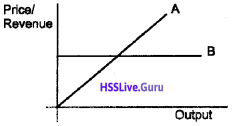1. Total revenue curve
2. Average/Marginal revenue curve

Question 10.
Choose the correct answer from the given multiple choices.
a. Identify the equilibrium condition of a firm under perfect competition.

1. AC=MR, & AC cuts MR from above.
2. MC=MR, & AC cuts MR from below.
3. AC=MR, & MC cuts MR from below.
4. MC=MR, &MC cuts MR from above.
5. MC=MR, & MC cuts MR from below.

b. The demand for the product of a firm is perfectly elastic in one of the following markets.
Identify the market.

1. monopoly
2. monopolistic competition
3. perfect competition
4. monopsony
5. oligopoly

a. 5
b. 3

### Plus Two Economics The Theory of The Firm Under Perfect Competition Three Mark Questions and Answers

Question 1.
Match the following.

 A B Perfect competition TR-TC TR Minimum point of AVC Profit Price taker Shutdown point Price x Quantity Supply curve of the firm Rise in-unit tax Shift of supply curve Rising portion of SMC

 A B Perfect competition Price taker TR Price x Quantity Profit TR-TC Shutdown point Minimum point of AVC Supply curve of the firm Rising portion of SMC Shift of supply curve Rise in-unit taxQuestion 2.
A firm maximizes profit when the difference between total revenue and total cost is the maximum. Profit is maximized when certain conditions are satisfied. Do you agree?
Yes.
A firm maximizes profit when the following three conditions are satisfied.

1. The market price, p, is equal to the marginal cost.
2. The marginal cost is nondecreasing.
3. In the short run, the market price must be greater than or equal to the average variable cost. In the long run, the market price must be grater than or equal to the average cost.

Question 3.
Write the economic terms

1. Cost vary with output
2. Total Cost divided by quantity
3. TRn – TRn-1
4. TR = TC point
5. A case where an increase in all the inputs lead to a just proportionate increase in output.

1. Variable cost
2. Average cost
3. Marginal revenue
4. Breakeven point
5. Constant returns

Question 4.
Perfect competition does not exist in the real world. Do you agree? Substantiate your view.
Yes, I agree to the statement that in the real world perfect competition does not exist.

This is because, it is rare to find the features of perfect competition especially the features like perfect knowledge, perfect mobility of factors and product, etc. What we really find in the world is monopolistic competition which is a mix of perfect competition and monopoly.

Question 5.
A firm’s supply curve in the short run is the rising part of the SMC curve. Why?
A firm under perfect competition in the short run will start supplying only if the price is equal to or greater than the short run average variable cost. Therefore, the rising part of the short run marginal cost which begins with the minimum SAVC is the supply curve of the firm in the short run.

Question 6.
Imagine that S° is the original supply curve of the firm. If a unit tax is imposed, what happens to the supply curve? Show the change in a diagram.
If a unit tax is imposed, firm’s long run supply curve shifts to the left. It is shown in the following diagram.Question 7.
Fill in the blanks.

1. Long run price under perfect competition will be equal to ……………….
2. Maximum price fixed for a product by the government is called ………..
3. The point denoted by the minimum of AVC is called …………

1. Average cost
2. Price ceiling
3. Shutdown pointQuestion 8.
a. A fall in supply caused by a fall in price is known as:

1. Contraction of supply
2. Expansion of supply
3. Increase in supply ‘

b. When supply curve is a vertical straight line, supply is

1. Perfectly elastic
2. Perfectly inelastic
3. Unitary elastic

c. The price under perfect competition. short run should be at least equal to

1. Short run MC
2. Short run AC
3. Short run AVC

a. Contraction of supply
b. Perfectly inelastic
c. Short run AVC

Question 9.
List the factors affecting elasticity of supply.
The factors affecting elasticity of supply are

1. nature of the commodity
2. cost of production
3. time period
4. technique of production

Question 10.
Observe the following figures and answer the questions.1. Point out the elasticities on the above supply curves.
2. Which method is applied here?

1. supply curves:

• S1 Elastic supply
• S2 Unitary elastic supply
• S3 Inelastic supply

2. Geometric method is applied here.Question 11.

1. Identify the market structure that is represented by their curve in the diagram.
2. Explain why the AR curve is horizontal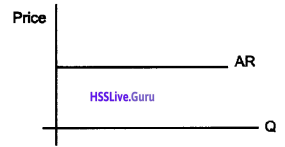1. Perfect competition

2. It is assumed that under perfect competition compared to the industry the share of each firm is meager. No firm can influence the market supply. So even if a firm doubles the quantity supplied the market supply will not change. The price remains the same. So theARorthe demand curve is horizontal.

Question 12.
Information about a firm is given in the following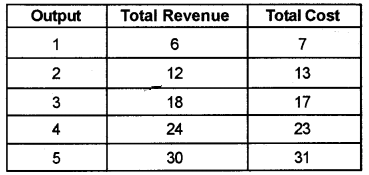Find out the equilibrium level of output in terms of MC & MR. Give reasons for your answer.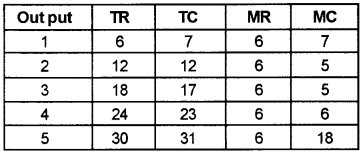Equilibrium quantity is 4. At this level of output MC = MR & MC cuts MR from below.

Question 13.
A firm can sell any quantity of the output it produces at a given price. If so, what is the behaviour of marginal revenue and average revenue? Draw the two curves in a single diagram.
The demand curve facing the firm is perfectly elastic. At this condition AR=MR=Price### Plus Two Economics The Theory of The Firm Under Perfect Competition Five Mark Questions and Answers

Question 1.
Consider the following table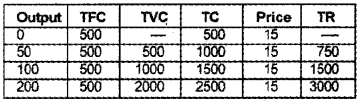1. At which production level there will be no profit or loss to the producer?
2. Comment on the profit and loss conditions as TC 1000 and TR750.

1. At the production level of 100 units of output, the producer incurs 1500 TC and 1500 TR. So there will be no profit or loss to the producer

2. When TC =1000
TR = 750
Loss = TC-TR
= 1000-750 = 250Question 2.
How would each of the following affect the market supply curve for wheat?

1. A new and improved technique is discovered.
2. The price of fertilizers falls
3. The government offers new tax concessions to farmers
4. Bad weather affects the crops.

1. A new and improved technique is discovered: increase market supply
2. The price of fertilizers falls: increase market supply
3. The government offers new tax concessions to farmers: increase market supply
4. Bad weather affects the crops: decrease market supply

Question 3.
The following table shows the total cost schedule of a competitive firm. It is given that the price of the good is ₹10. Calculate the profit at each output level. Find the profit maximizing level of output.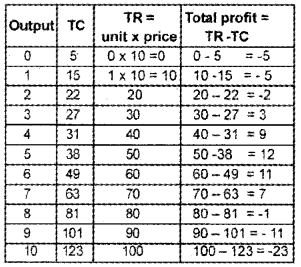Question 4.
Explain briefly break even point with the help of an example.
XUS Break even point refers to a situation when total revenue is equal to total cost assuming a given selling price per unit of output.
TR = TC
This can be explained with the help of an example.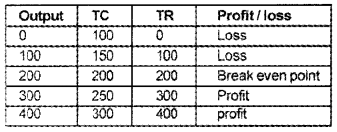If the firm produces less than 200 units, itsTR< TC. So it bears loss. If the firm produces more than 200 units, its TR > TC. So it earns profit.

If the firm produces 200 units, its TR = TC. This is the breakeven point. Break even point is a normal profit point as beyond it the firm earns super normal profits and below it, the firm incur losses.

Question 5.
a. profit of a firm is the revenue earned:

1. zero of cost
2. net of cost
3. gross of cost
4. none of these

b. TMC curve cuts LAC curve :

1. at minimum point
2. at maximum point
3. below the LAC curve
4. none of these

c. under perfect competition, firm is :

1. price taker
2. price maker
3. both 1 and 2
4. none of the above

d. MR can be negative but AR is:

1. negative
2. positive
3. either positive or negative
4. none of the above

a. net of cost
b. at minimum point
c. price taker
d. positiveQuestion 6.
Observe the following diagram.Plot the break even point and shut down point.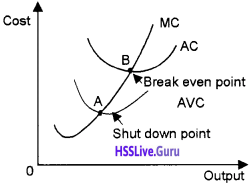• Point ‘A’ represents shut down point
• Point ‘B’ represents the break even point.

Question 7.
Complete the following tables and identify the market structure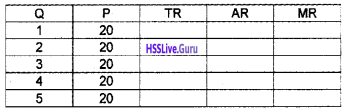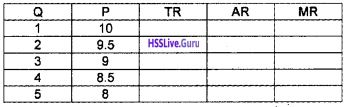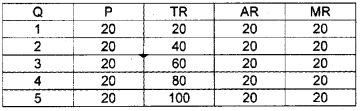This market represents a perfectly competitive market because in this market, P = AR = MR.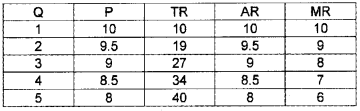This market represents a monopoly market. In this market, AR and MR are different and AR > MR.

Question 8.
At the market price of ₹10, a firm supplies 4 units of orange. The market price rises to ₹30. The price elasticity of the firm’s supply is 1.25. What quantity will the firm supply at the new price?
In the given example,
P = 10 Q = 4 P1 = 30 es = 1.25
ΔP = 30-10 = 20
Applying these values in the formula, we get, $$e_{s}=\frac{\Delta Q}{\Delta P} \times \frac{P}{Q}$$ΔQ =1.25×8=10

Question 9.
Suppose that the market demand in a perfectly competitive industry is given by, Qd = 7000 – 500 p and the market supply function is given by, Qs = 4000 +250 P. Find the market equilibrium price.
Equilibrium is determined by the condition,
Qd = Qs.
In this example,
7000-500 p = 4000 +250 p
7000-4000 = 250 p + 500 p
3000 = 750 P
∴ $$P=\frac{3000}{750}=4$$
Therefore, the equilibrium price in the market is ₹4.
7000 – 500 p = 4000 + 250 p
7000 – 4000 = 250 p + 500 p
3000 = 750 P
∴ $$P=\frac{3000}{750}=4$$Question 10.
Point out the features of perfect competition.
Features of perfect competion are pointed out below.

1. Large number of buyers and sellers
2. Homogeneous product
3. Freedom of entry and exit
4. Free movement of product and factors of production.
5. Profit motive.
6. Perfect knowledge of market conditions
7. Absence of transport cost.

Question 11.
Identify from the diagram below.

1. Shutdown point and breakeven point.
2. Distinguish between these two.1. c is the break even point. b is the shut even point

2. Break down point shows a situation where a firm earns no profit or no loss. It is the point where AR = AC. Shut down point shows a situation where a firm is compelled to stop the production since it is not able to cover its variable cost. This is the situation where the firm’s P > AVC.

Question 12.
Identify the profit maximizing level of output from the diagram below. List out the condition for profit maximization.b is the profit maximizing level of output.
MR = MC
At the profit maximizing level of output MC is non decreasing, that is the slope of MC is positive.
P > AVC.

Question 13.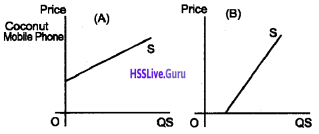a. Mobile Phone

b. Coconut
The supply of mobile phones are relatively price elastic. This is because the purchase of mobile phones can easily react to a change in price. It is a manufactured good. All the raw materials needed to produce a mobile phone can easily be available. The producers can pile up stock and when price increases they can increase the supply.

The supply of coconut can be price inelastic. This is because the producers cannot easily react to a change in the price of coconut. Years needed for a coconut tree to get mature and start to produce coconuts. It is an agricultural product. The producers cannot hold the stock of coconut fora longer duration.Question 14.
The following table gives you certain information about a firm.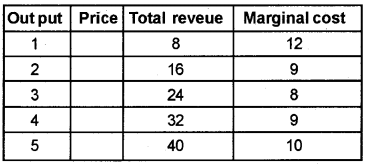1. Find the price at which output is sold and identify the form market,
2. Is this firm a price-taker or price-maker? Give reasons.
3. Find the firm’s equilibrium level of output in terms of MC &MR. Give reasons.
4. Also find profit of the firm at this level of output.

1. Price = 8
2. Price-taker, a firm has no influence on price determination under perfect competition.
3. Equilibrium quantity is 3. At this level of output MC=MR.
4. Firm is at no profit-no loss condition, i. e., he is at break even point.

### Plus Two Economics The Theory of The Firm Under Perfect Competition Eight Mark Questions and Answers

Question 1.
Prepare a seminar report on ‘perfect competitive markets’
Respected teachers and dear friends,
The topic of my seminar paper is perfect competitive markets. The term market refers to all the places in which buyers and sellers are in contact with each other for the purchase and sale of any commodity or service. There are different kinds of markets based on their characteristics – say perfect competitive markets and noncompetitive markets.

Introduction:
Perfect competition is a market situation characterized by the existence of large number of buyers and sellers, homogeneous products, free mobility of factors of production, freedom of entry and exit, perfect knowledge of market conditions and absence of transportation costs.

Contents:

1. Profit Maximisation
2. Profit Maximisation in short-run: Diagrammatic representation
3. Long run profit maximisation

1. Profit Maximisation:
The main objective of the producer is to maximize the profit levels of his firm. The output level at which the firm maximizes the profit is called the equilibrium of the firm. The profit level of the firm is the difference between Total Revenue and Total Cost. Symbolically it is represented as X = TR – TC.

The firm under perfect competition maximizes its profit under three conditions. They are:

• The MC must be equal to MR (MC = MR)
• MC must be non-decreasing. It means that MC curve should cut the MR from below.
• Third condition has two parts, one for short-term and the other for long-term.

a. In the short run, price should be more than or equal to the minimum point of Average Variable Cost (AVC). It can be denoted as P> AVC.

b. In the long run, price should be more than or equal to the minimum point of Average Cost (AC). It can be denoted as P > AC.

2. Profit Maximisation in short run: Diagrammatic representation:
The profit maximizing condition of a firm in short-run can be understood from the figure. All the three profit maximizing conditions of a firm in short run are satisfied at point of output level q0.

• The price must be equal to MC (P = MC)
• MC must be non-decreasing.
• P > AVC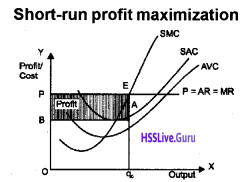All the profit maximizing conditions are satisfied in the figure given above.

1. The prices become equal to MC. At point E, the firm reaches equilibrium at the output level q (MC = MR).
2. At q MC is non-decreasing at point E.
3. At point E, prices have become more than AVC (P > AVC at point E).

Therefore, three conditions for the equilibrium level of output are depicted in a single figure.

3. Long run profit maximisation:
The profit maximization level of the firm is reached when the long run supply curve of the firm is that portion of LRMC curve which lies over and above the minimum point of LRAC curve (P > minimum of LRAC). The supply curve of a firm, in the long run, is the rising portion of minimum point of LMRC. It can be explained with the help of the following figure.Conclusion:
Thus it can be concluded that perfect competition is a market structure characterized by complete absence rivalry among individual sellers. Sellers in the market do not hold any freedom of influencing market prices. Therefore, they are known as price takers. However, it should be admitted that it is a rare form of market.Question 2.
The diagram shows one of the short run equilibrium situations of a firm under perfect competition.

1. List out any four features of such market condition.
2. Identify the equilibrium situation of the firm profit, normal profit, loss diagrammatically. Show a situation in which the firm makes a profit.
3. With the help of a diagram explain the long run situation of a firm under perfect competition.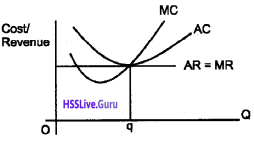1. Market Conditions:

• Large number of sellers
• Homegeneous product
• Firm is a price taker
• Free entry and exit

2. The firm is making a normal profit.
The firm produces ‘oq’ level of output and charges a price ‘op’. The shaded area in the diagram shows profit.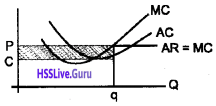3. Firm under perfeert competition in the long run makes only normal profit. This is because if firms are making profit, new entrants will be attracted into the industry.

The price falls due to increase in supply and the extra profit will be taken away. If the firms are making loss some of them will leave the industry, price rises and the loss will be turned into profit. This is shown in the diagram below.

The firm under perfect competition in the long run will produce ‘oq’ level of output and charges a price ‘op’.Question 3.
How are price and output determined under perfect competition in the short run? Compare the profit of a firm in the short run and long run and bring out the difference. Give suitable diagram.
Under perfect competition, market determines the price – price taker and not price maker firms produce the output that maximises its profit – a firm may get abnormal profit or normal profit or loss in the short run – only normal profit will prevail in the long run – due to free entry and exit – Draws separate diagram for short run and long run-explains price and output determination as well as profit in short run and long run.

PROFIT MAXIMIZATION IN SHORT-RUN:
DIAGRAMMATIC REPRESENTATION:
The profit maximizing condition of a firm in short-rn can be understood from the figure. All the three profit maximizing conditions of a firm in short run are satisfied at point of output level q0.

1. The price must be equal to MC (P = MC)
2. MC must be non-decreasing.
3. P > AVC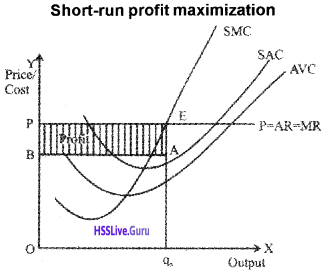All the profit maximizing conditions are satisfied in the figure given above.

1. The prices become equal to MC. At point E, the firm reaches equilibrium at the output level q (MC = MR). 0
2. At q MC is non-decreasing at point E.
3. At point E, prices have become more than AVC (P > AVC at point E).

Therefore, three conditions for the equilibrium level of output are depicted in a single figure.

LONG RUN PROFIT MAXIMISATION:
The profit maximization level of the firm is reached when the long run supply curve of the firm is that portion of LRMC curve which lies over and above the minimum point of LRAC curve (P>minimum of LRAC). The supply curve of a firm, in the long run, is the rising portion of minimum point of LMRC. It can be explained with the help of the following figure.